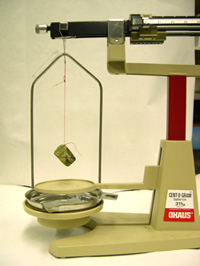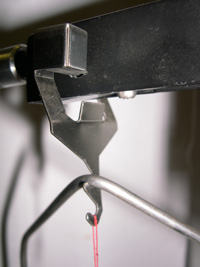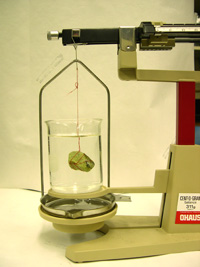## Summary

The purpose of this lab is to tie together several previous lectures and laboratory experiments by asking a large scale question that can be answered with simple tools. Students are asked to answer the following question:

What happens at convergent plate boundaries where oceanic lithosphere collides with continental lithosphere (and why)?

The answer is based on laboratory measurements and calculations of the specific gravity of common rocks and minerals.

Used this activity? Share your experiences and modifications

## Learning Goals

• Students apply results gathered during the laboratory to answer questions regarding subduction.
• Students learn to use a quadruple beam balance.
• Students write critically and are required to use information gleaned from numerous previous classes.

## Context for Use

This is a laboratory activity that draws on previous lectures. In order for students to complete this lab, they must have been exposed to the following topics: The student lab report must contain the following topics (previously discussed):
• forces that may drive plate tectonics (e.g., convection, gravity, etc.)
• oceanic lithosphere vs. continental lithosphere (composition)
• rock types associated with the two types of lithosphere
• mineral assemblages and chemistry
• sources of error (lab methods)
• convergent plate boundaries (including faults, benioff zones, subduction, etc.)
• atomic mass, subatomic particles
• iron catastrophe
• specific gravity, density, isostasy
The write-up for this activity requires the student to really think about all of the concepts discussed in previous weeks. The laboratory component itself is not difficult, but synthesizing their results and combining it with prior knowledge makes the write-up challenging, yet very rewarding.

## Description and Teaching Materials

Required equipment: Ohaus Cent-O-Gram Balance, thread, gabbro, basalt, granite, rhyolite, the common minerals on Bowen's Reaction Series, galena, 50 ml beaker, calculator, and water.

To answer the question posed at the beginning of lab [What happens at convergent plate boundaries where oceanic lithosphere collides with continental lithosphere (and why)?], we assume that continental lithosphere is felsic and oceanic lithosphere is mafic. Students measure the specific gravity of gabbro, basalt, granite, rhyolite, and the common minerals on Bowen's Reaction Series (include galena because of its high density). Start lab with a discussion of density and make it clear that the error one would get using displacement to measure volume (on small samples) would make the activity ineffective. Describe specific gravity and show the students how to calculate this value using the following equation:

Specific gravity = Massair / ( Massair - Masswater)

Make note that although specific gravity is a unitless number, the magnitude of that number corresponds directly to the units of density (g/cm3). The Massair is measured once the mineral is tied to a string (Figure 1) and suspended from a balance (Figure 2).Figure 1. Mass in air.Figure 2. Sample tied to balance.Figure 3. Mass in water.

The Masswater is measured when the mineral is completely submerged, but floating, in water (Figure 3).

Be sure to use large books of mica; the surface tension on small books of mica generally leads to erroneous results.

## Teaching Notes and Tips

Divide the students in lab into groups of two; each group has to determine the specific gravity of five rocks or minerals. Be sure that all rocks and minerals are measured and that several groups measure the specific gravity of the same type of sample. It should take about one hour for the students to tie the minerals to a piece of thread, measure the masses (to hundredths of a gram), and calculate the specific gravity. Students need to use signficant figures and learn how to round the values on a quadruple beam balance.

Make a table on the board that lists the minerals (in order of Bowen's Reaction Series) and the four rocks. Ask the students to report their results for each sample. Discuss the range of values acquired (talk about purity, end-member composition, error...). Put mineral formulae and the values of density reported by Deer, Howie, and Zussman along side the student results and discuss the similarities (or see the attached file (Excel PRIVATE FILE 19kB Jul12 06)).

Once the discussion ceases, hand out the assignment. It might be appropriate to develop a grading rubric for this activity during the class, or use the one associated with the assignment (Microsoft Word PRIVATE FILE 36kB Jul12 06).

The answer should be stated in the following form: Oceanic lithosphere is more dense than continental lithosphere because the rocks that make up the oceanic lithosphere (gabbro and basalt) contain minerals that are dense (olivine, pyroxene, and Ca-rich plagioclase feldspar) because those minerals contain dense elements (iron, magnesium), that is, those that have high atomic masses.

## Assessment

See the grading rubric associated with the assignment.

## References and Resources

The assignment (Microsoft Word PRIVATE FILE 36kB Jul12 06)
Mineral formulae and densities (Excel PRIVATE FILE 19kB Jul12 06)